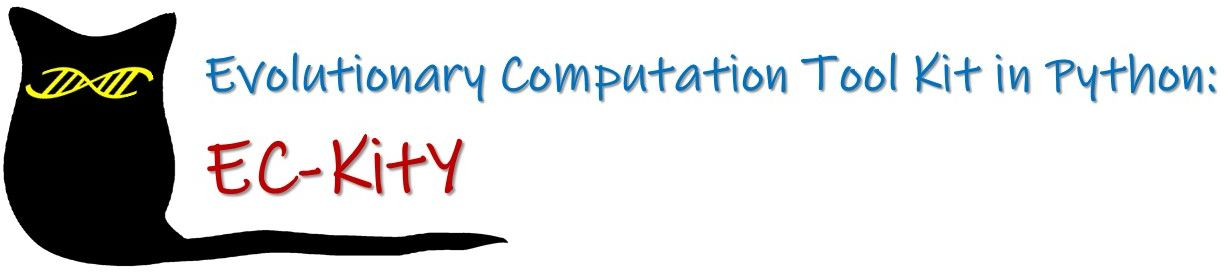{{ message }}

## EC-KitY / EC-KitY Public

EC-KitY is a scikit-learn-compatible Python tool kit for doing evolutionary computation.

Switch branches/tags
Nothing to show

## Latest commit

`d586139`

## Files

Failed to load latest commit information.
Type
Name
Commit timeEC-KitY is a Python tool kit for doing evolutionary computation. It is scikit-learn-compatible and is distributed under the GNU General Public License v3.0. Currently we have implemented tree-based genetic programming (GP), but EC-KitY will grow!

EC-KitY is:

• A comprehensive toolkit for running evolutionary algorithms
• Written in Python
• Can work with or without scikit-learn, i.e., supports both sklearn and non-sklearn modes
• Designed with modern software engineering in mind
• Designed to support all popular EC paradigms (GA, GP, ES, coevolution, multi-objective, etc').

### Dependencies

For the basic evolution mode, EC-KitY requires:

• numpy (>=1.14.6)
• pandas (>=0.25.0)
• overrides (>= 6.1.0)

For sklearn mode, EC-KitY additionally requires:

• scikit-learn (>=0.24.2)

### User installation

`git clone https://github.com/EC-KitY/EC-KitY.git`

For basic package installation: `pip install -r requirements.txt`

### Documentation

After cloning the project, navigate to the `doc\api` folder and open `eckity.html` file. This will open an html page from which you can access the documentation of all the modules.

(Work in progress - some modules and functions are not documented yet.)

### Tutorials

There are 4 tutorials available here, walking you through running EC-KitY both in sklearn mode and in non-sklearn mode.

### Examples

More examples are in the examples folder. All you need to do is define a fitness-evaluation method, through a `SimpleIndividualEvaluator` sub-class.

### Basic example (no sklearn)

You can run an EA with just 3 lines of code. The problem being solved herein is simple symbolic regression.

Additional information on this problem can be found in the Symbolic Regression Tutorial.

```from eckity.algorithms.simple_evolution import SimpleEvolution
from eckity.subpopulation import Subpopulation
from examples.treegp.non_sklearn_mode.symbolic_regression.sym_reg_evaluator import SymbolicRegressionEvaluator

algo = SimpleEvolution(Subpopulation(SymbolicRegressionEvaluator()))
algo.evolve()
print(f'algo.execute(x=2,y=3,z=4): {algo.execute(x=2, y=3, z=4)}')```

### Example with sklearn

The problem being solved herein is the same problem, but in this case we also involve sklearn compatability - a core feature of EC-KitY. Additional information for this example can be found in the Sklearn Symbolic Regression Tutorial.

A simple sklearn-compatible EA run:

```from sklearn.datasets import make_regression
from sklearn.metrics import mean_absolute_error
from sklearn.model_selection import train_test_split

from eckity.algorithms.simple_evolution import SimpleEvolution
from eckity.creators.gp_creators.full import FullCreator
from eckity.genetic_encodings.gp.tree.utils import create_terminal_set
from eckity.sklearn_compatible.regression_evaluator import RegressionEvaluator
from eckity.sklearn_compatible.sk_regressor import SKRegressor
from eckity.subpopulation import Subpopulation

X, y = make_regression(n_samples=100, n_features=3)
terminal_set = create_terminal_set(X)

algo = SimpleEvolution(Subpopulation(creators=FullCreator(terminal_set=terminal_set),
evaluator=RegressionEvaluator()))
regressor = SKRegressor(algo)

X_train, X_test, y_train, y_test = train_test_split(X, y, test_size=0.2)
regressor.fit(X_train, y_train)
print('MAE on test set:', mean_absolute_error(y_test, regressor.predict(X_test)))```

### Feature comparison

We are working on a paper that describes EC-KitY. For now, here is a table comparing EC-KitY with 8 other libraries:### Authors

Moshe Sipper, Achiya Elyasaf, Itai Tzruia, Tomer Halperin

### Citation

Citations are always appreciated 😊:

``````@article{eckity2022,
author = {Sipper, Moshe and Halperin, Tomer and Tzruia, Itai and  Elyasaf, Achiya},
title = {{EC-KitY}: Evolutionary Computation Tool Kit in {Python}},
journal = {},
volume = {},
pages = {},
year = {2022},
note = {in preparation}
}

@misc{eckity2022git,
author = {Sipper, Moshe and Halperin, Tomer and Tzruia, Itai and  Elyasaf, Achiya},
title = {{EC-KitY}: Evolutionary Computation Tool Kit in {Python}},
year = {2022},
publisher = {GitHub},
journal = {GitHub repository},
howpublished = {\url{https://www.eckity.org/} }
}

``````

EC-KitY is a scikit-learn-compatible Python tool kit for doing evolutionary computation.

1 tags

## Packages 0

No packages published

•
•
•
•